# McGraw Hill Math Grade 6 Lesson 3.2 Answer Key Estimating Quotients

Practice questions available in McGraw Hill Math Grade 6 Answer Key PDF Lesson 3.2 Estimating Quotients will engage students and is a great way of informal assessment.

Exercises
Estimate

Question 1.
146 ÷ 12
146 divided by 12, we get the quotient 12.16.Explanation:
Quotient of 146 ÷ 12, we get 12.16.

Question 2.
244 ÷ 8
244 divided by 8, we get the quotient 30.5.Explanation:
Quotient of 244 ÷ 8, we get 30.5.

Question 3.
91 ÷ 9
91 divided by 9, we get the quotient 10.11.Explanation:
Quotient of 91 ÷ 9, we get 10.11.

Question 4.
399 ÷ 13
399 divided by 13, we get the quotient 30.69.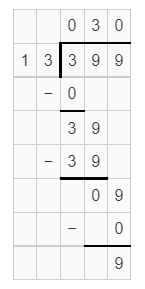Explanation:
Quotient of 399 ÷ 13, we get 30.69.

Question 5.
412 ÷ 25
412 divided by 25, we get the quotient 16.48.Explanation:
Quotient of 412 ÷ 25, we get 16.48.

Question 6.
1321 ÷ 13
1321 divided by 13, we get the quotient 101.61.Explanation:
Quotient of 1321 ÷ 13, we get 101.61.

Question 7.
447 ÷ 9
477 divided by 9, we get the quotient 53.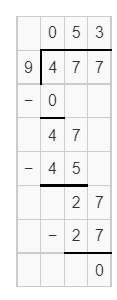Explanation:
Quotient of 447 ÷ 9, we get 53.

Question 8.
672 ÷ 33
672 divided by 33, we get the quotient 20.36.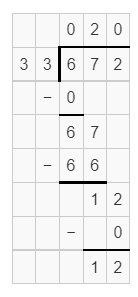Explanation:
Quotient of 672 ÷ 33, we get 20.36.

Question 9.
441 ÷ 91
441 divided by 91, we get the quotient 4.84.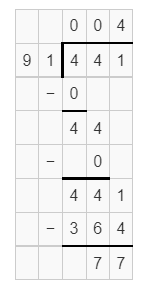Explanation:
Quotient of 441 ÷ 91, we get 4.84.

Question 10.
252 ÷ 23
252 divided by 23, we get the quotient 10.95.Explanation:
Quotient of 252 ÷ 23, we get 10.95.

Question 11.
49 ÷ 4
49 divided by 4, we get the quotient 12.25.Explanation:
Quotient of 49 ÷ 4, we get 12.25.

Question 12.
3211 ÷ 80
3211 divided by 80, we get the quotient 40.13.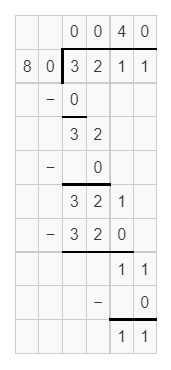Explanation:
Quotient of 3211 ÷ 80, we get 40.13.

Question 13.
1247 ÷ 50
1247 divided by 50, we get the quotient 24.94.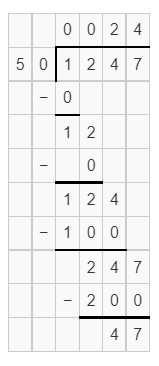Explanation:
Quotient of 1247 ÷ 50, we get 24.94.

Question 14.
333 ÷ 16
333 divided by 16, we get the quotient 20.81.Explanation:
Quotient of 333 ÷ 16, we get 20.81.

Question 15.
564 ÷ 11
564 divided by 11, we get the quotient 51.27.Explanation:
Quotient of 564 ÷ 11, we get 51.27.

Question 16.
233 ÷ 20
233 divided by 20, we get the quotient 11.65.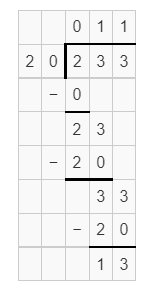Explanation:
Quotient of 233 ÷ 20, we get 11.65.

Question 17.
889 ÷ 45
889 divided by 45, we get the quotient 19.75.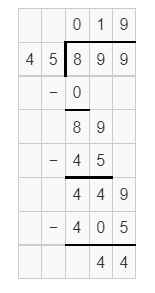Explanation:
Quotient of 889 ÷ 45, we get 19.75.

Question 18.
489 ÷ 45
489 divided by 45, we get the quotient 10.86.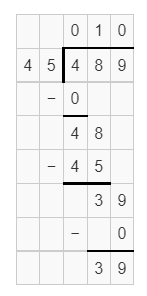Explanation:
Quotient of 489 ÷ 45, we get 10.86.

Question 19.
77 ÷ 5
77 divided by 5, we get the quotient 15.4.Explanation:
Quotient of 77 ÷ 5, we get 15.4.

Question 20.
732 ÷ 9